# Balbharati solutions for Physics 11th Standard Maharashtra State Board chapter 9 - Optics [Latest edition]

#### Chapters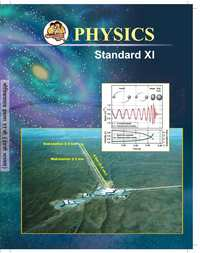## Chapter 9: Optics

ExercisesOthers
Exercises [Pages 184 - 187]

### Balbharati solutions for Physics 11th Standard Maharashtra State Board Chapter 9 Optics Exercises [Pages 184 - 187]

Exercises | Q 1. (i) | Page 184

Choose the correct option.

As per the recent understanding, light consists of

• rays

• waves

• corpuscles

• photons obeying the rules of waves

Exercises | Q 1. (ii) | Page 184

Choose the correct option.

Consider the optically denser lenses P, Q, R, and S drawn below. According to the Cartesian sign convention which of these have a positive focal length?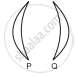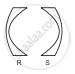• Only P

• Only P and Q

• Only P and R

• Only Q and S

Exercises | Q 1. (iii) | Page 184

Choose the correct option.

Two plane mirrors are inclined at angle of 40° between them. Number of images seen of a tiny object kept between them is

• Only 8

• Only 9

• 8 or 9

• 9 or 10

Exercises | Q 1. (iv) | Page 184

Choose the correct option.

A concave mirror of curvature 40 cm, used for shaving purposes produces image of double size as that of the object. Object distance must be

• 10 cm only

• 20 cm only

• 30 cm only

• 10 cm or 30 cm

Exercises | Q 1. (v) | Page 184

Choose the correct option.

Which of the following aberrations will NOT occur for spherical mirrors?

• Chromatic aberration

• Coma

• Distortion

• Spherical aberration

Exercises | Q 1. (vi) | Page 184

Choose the correct option.

There are different fish, monkeys, and water of the habitable planet of the star Proxima b. A fish swimming underwater feels that there is a monkey at 2.5 m on the top of a tree. The same monkey feels that the fish is 1.6 m below the water surface. Interestingly, height of the tree and the depth at which the fish is swimming are exactly same. Refractive index of that water must be

• 6/5

• 5/4

• 4/3

• 7/5

Exercises | Q 1. (vii) | Page 184

Choose the correct option.

Consider the following phenomena/ applications: P) Mirage, Q) rainbow, R) Optical fibre and S) glittering of a diamond. Total internal reflection is involved in

• Only R and S

• Only R

• Only P, R and S

• all the four

Exercises | Q 1. (viii) | Page 184

Choose the correct option.

A student uses spectacles of number -2 for seeing distant objects. Commonly used lenses for her/his spectacles are

• bi-concave

• double concave

• concavo-convex

• convexo-concave

Exercises | Q 1. (ix) | Page 185

Choose the correct option.

A spherical marble, of refractive index 1.5 and curvature 1.5 cm, contains a tiny air bubble at its centre. Where will it appear when seen from outside?

• 1 cm inside

• at the centre

• 5/3 cm inside

• 2 cm inside

Exercises | Q 1. (x) | Page 185

Choose the correct option.

Select the WRONG statement.

• Smaller angle of prism is recommended for greater angular dispersion.

• Right-angled isosceles glass prism is commonly used for total internal reflection.

• Angle of deviation is practically constant for thin prisms.

• For emergent rays to be possible from the second refracting surface, certain minimum angle of incidence is necessary from the first surface.

Exercises | Q 1. (xi) | Page 185

Choose the correct option.

Angles of deviation for extreme colours are given for different prisms. Select the one having maximum dispersive power of its material.

• 7°, 10°

• 8°, 11°

• 12°, 16°

• 10°, 14°

Exercises | Q 1. (xii) | Page 185

Choose the correct option.

Which of the following is not involved in formation of a rainbow?

• refraction

• angular dispersion

• angular deviation

• total internal reflection

Exercises | Q 1. (xiii) | Page 185

Choose the correct option.

Consider the following statements regarding a simple microscope:

(P) It allows us to keep the object within the least distance of distant vision.

(Q) Image appears to be biggest if the object is at the focus.

(R) It is simply a convex lens.

• Only (P) is correct

• Only (P) and (Q) are correct

• Only (Q) and (R) are correct

• Only (P) and (R) are correct

Exercises | Q 2. (i) | Page 185

As per recent development, what is the nature of light? Wave optics and particle nature of light are used to explain which phenomena of light respectively?

Exercises | Q 2. (ii) (a) | Page 185

Which phenomena can be satisfactorily explained using ray optics?

Exercises | Q 2. (ii) (b) | Page 185

State the assumptions on which ray optics is based.

Exercises | Q 2. (iii) | Page 185

What is focal power of a spherical mirror or a lens? What may be the reason for using P = 1/"f" as its expression?

Exercises | Q 2. (iv) | Page 185

At which positions of the objects do spherical mirrors produce a diminished image?

Exercises | Q 2. (iv) | Page 185

At which positions of the objects do spherical mirrors produce a magnified image?

Exercises | Q 2. (v) | Page 185

State the restrictions for having images produced by spherical mirrors to be appreciably clear.

Exercises | Q 2. (vi) | Page 185

Explain spherical aberration for spherical mirrors. How can it be minimized? Can it be eliminated by some curved mirrors?

Exercises | Q 2. (vii) | Page 185

Define absolute refractive index and relative refractive index. Explain in brief with an illustration for each.

Exercises | Q 2. (viii) | Page 185

Explain ‘mirage’ as an illustration of refraction.

Exercises | Q 2. (ix) | Page 185

Under what conditions are total internal reflection possible? Explain it with a suitable example.

Exercises | Q 2. (ix) | Page 185

Define the critical angle of incidence and obtain an expression for it.

Exercises | Q 2. (x) | Page 185

Describe the construction and working of an optical fibre.

Exercises | Q 2. (x) | Page 185

What are the advantages of optical fibre communication over electronic communication?

Exercises | Q 2. (xi) | Page 185

Why is prism binoculars preferred over traditional binoculars? Describe its working in brief.

Exercises | Q 2. (xii) | Page 185

A spherical surface separates two transparent media. Derive an expression that relates object and image distances with the radius of curvature for a point object. Clearly state the assumptions, if any.

Exercises | Q 2. (xiii) | Page 186

Derive lens makers’ equation. Why is it called so? Under which conditions focal length f and radii of curvature R are numerically equal for a lens?

Exercises | Q 3. (i) | Page 186

Answer the following question in detail.

What are the different types of dispersions of light? Why do they occur?

Exercises | Q 3. (ii) | Page 186

Answer the following question in detail.

Define angular dispersion for a prism.

Exercises | Q 3. (ii) | Page 186

Answer the following question in detail.

Obtain angular dispersion expression for a thin prism. Relate it with the refractive indices of the material of the prism for corresponding colours.

Exercises | Q 3. (iii) | Page 186

Answer the following question in detail.

Explain and define the dispersive power of transparent material. Obtain its expressions in terms of angles of deviation and refractive indices.

Exercises | Q 3. (iv) (i) | Page 186

Answer the following question in detail.

State the conditions under which a rainbow can be seen.

Exercises | Q 3. (iv) (ii) | Page 186

Answer the following question in detail.

Explain the formation of a primary rainbow. For which angular range with the horizontal is it visible?

Exercises | Q 3. (iv) (iii) | Page 186

Answer the following question in detail.

Explain the formation of a secondary rainbow. For which angular range with the horizontal is it visible?

Exercises | Q 3. (iv) (iv) | Page 186

Answer the following question in detail.

Is it possible to see primary and secondary rainbow simultaneously? Under what conditions?

Exercises | Q 3. (v) (i) | Page 186

Answer the following question in detail.

Explain chromatic aberration for spherical lenses. State a method to minimize or eliminate it.

Exercises | Q 3. (v) (ii) | Page 186

Answer the following question in detail.

What is achromatism? Derive a condition to achieve achromatism for a lens combination. State the conditions for it to be converging.

Exercises | Q 3. (vi) | Page 186

Answer the following question in detail.

Describe spherical aberration for spherical lenses. What are the different ways to minimize or eliminate it?

Exercises | Q 3. (vii) | Page 186

Answer the following question in detail.

Define and describe the magnifying power of an optical instrument.

Exercises | Q 3. (vii) | Page 186

Answer the following question in detail.

How does magnifying power differ from linear or lateral magnification?

Exercises | Q 3. (viii) | Page 186

Answer the following question in detail.

Derive an expression for the magnifying power of a simple microscope. Obtain its minimum and maximum values in terms of its focal length.

Exercises | Q 3. (ix) | Page 186

Answer the following question in detail.

Derive the expressions for the magnifying power and the length of a compound microscope using two convex lenses.

Exercises | Q 3. (x) | Page 186

Answer the following question in detail.

What is a terrestrial telescope and an astronomical telescope?

Exercises | Q 3. (xi) | Page 186

Answer the following question in detail.

Obtain the expressions for magnifying power and the length of an astronomical telescope under normal adjustments.

Exercises | Q 3. (xii) (i) | Page 186

Answer the following question in detail.

What is the limitation in increasing the magnifying powers of a simple microscope?

Exercises | Q 3. (xii) (ii) | Page 186

Answer the following question in detail.

What is the limitation in increasing the magnifying powers of a compound microscope?

Exercises | Q 3. (xii) (iii) | Page 186

Answer the following question in detail.

What is the limitation in increasing the magnifying powers of the astronomical telescope?

Exercises | Q 4. (i) | Page 186

Solve Numerical example.

A monochromatic ray of light strike the water (n = 4/3) surface in a cylindrical vessel at angle of incidence 53°. Depth of water is 36 cm. After striking the water surface, how long will the light take to reach the bottom of the vessel? [Angles of the most popular Pythagorean triangle of sides in the ratio 3:4:5 are nearly 37°, 53°, and 90°]

Exercises | Q 4. (ii) | Page 186

Solve Numerical example.

Estimate the number of images produced if a tiny object is kept in between two plane mirrors inclined at 35°, 36°, 40° and 45°.

Exercises | Q 4. (iii) | Page 186

Solve Numerical example.

A rectangular sheet of length 30 cm and breadth 3 cm is kept on the principal axis of a concave mirror of focal length 30 cm. Draw the image formed by the mirror on the same diagram, as far as possible on scale.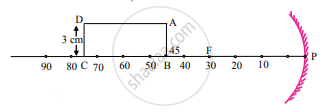Exercises | Q 4. (iv) | Page 186

Solve Numerical example.

A car uses a convex mirror of curvature 1.2 m as its rear-view mirror. A minibus of cross-section 2.2 m × 2.2 m is 6.6 m away from the mirror. Estimate the image size.

Exercises | Q 4. (v) | Page 187

Solve Numerical example.

A glass slab of thickness 2.5 cm having refractive index 5/3 is kept on an ink spot. A transparent beaker of very thin bottom, containing water of refractive index 4/3 up to 8 cm, is kept on the glass block. Calculate apparent depth of the ink spot when seen from the outside air.

Exercises | Q 4. (vi) | Page 187

Solve Numerical example.

A convex lens held some distance above a 6 cm long pencil produces its image of SOME size. On shifting the lens by a distance equal to its focal length, it again produces the image of the SAME size as earlier. Determine the image size.

Exercises | Q 4. (vii) | Page 187

Solve Numerical example.

Figure below shows the section ABCD of a transparent slab. There is a tiny green LED light source at the bottom left corner B. A certain ray of light from B suffers total internal reflection at nearest point P on the surface AD and strikes the surface CD at point Q. Determine refractive index of the material of the slab and distance DQ. At Q, the ray PQ will suffer partial or total internal reflection? [Angles of the most popular Pythagorean triangle of sides in the ratio 3:4:5 are nearly 37°, 53°, and 90°]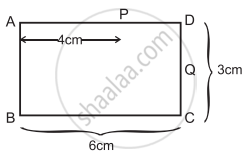Exercises | Q 4. (viii) | Page 187

Solve Numerical example.

A point object is kept 10 cm away from one of the surfaces of a thick double convex lens of refractive index 1.5 and radii of curvature 10 cm and 8 cm. Central thickness of the lens is 2 cm. Determine location of the final image considering paraxial rays only.

Exercises | Q 4. (ix) | Page 187

Solve Numerical example.

A monochromatic ray of light is incident at 37° on an equilateral prism of refractive index 3/2. Determine angle of emergence and angle of deviation. If angle of prism is adjustable, what should its value be for emergent rays to be just possible for the same angle of incidence?

Q 4. (x) | Page 187

Solve Numerical example.

From the given data set, determine angular dispersion by the prism and dispersive power of its material for extreme colours. nR = 1.62 nV = 1.66, δR = 3.1°

Exercises | Q 4. (xi) | Page 187

Solve Numerical example.

Refractive index of a flint glass varies from 1.60 to 1.66 for visible range. Radii of curvature of a thin convex lens are 10 cm and 15 cm. Calculate the chromatic aberration between extreme colours.

Exercises | Q 4. (xii) | Page 187

Solve Numerical example.

A person uses spectacles of ‘number’ 2.00 for reading. Determine the range of magnifying power (angular magnification) possible. It is a concavoconvex lens (n = 10.5) having a curvature of one of its surfaces to be 10 cm. Estimate that of the other.

Exercises | Q 4. (xiii) | Page 187

Solve Numerical example.

Focal power of the eye lens of a compound microscope is 6 dioptre. The microscope is to be used for maximum magnifying power (angular magnification) of at least 12.5. The packing instructions demand that length of the microscope should be 25 cm. Determine minimum focal power of the objective. How much will its radius of curvature be if it is a biconvex lens of n = 1.5?

## Chapter 9: Optics

ExercisesOthers## Balbharati solutions for Physics 11th Standard Maharashtra State Board chapter 9 - Optics

Balbharati solutions for Physics 11th Standard Maharashtra State Board chapter 9 (Optics) include all questions with solution and detail explanation. This will clear students doubts about any question and improve application skills while preparing for board exams. The detailed, step-by-step solutions will help you understand the concepts better and clear your confusions, if any. Shaalaa.com has the Maharashtra State Board Physics 11th Standard Maharashtra State Board solutions in a manner that help students grasp basic concepts better and faster.

Further, we at Shaalaa.com provide such solutions so that students can prepare for written exams. Balbharati textbook solutions can be a core help for self-study and acts as a perfect self-help guidance for students.

Concepts covered in Physics 11th Standard Maharashtra State Board chapter 9 Optics are Introduction to Optics, Nature of Light, Ray Optics Or Geometrical Optics, Reflection, Refraction, Refraction at a Spherical Surface and Lenses, Dispersion of Light and Prisms, Some Natural Phenomena Due to Sunlight, Defects of Lenses (Aberrations of Optical Images), Optical Instruments, Total Internal Reflection.

Using Balbharati 11th solutions Optics exercise by students are an easy way to prepare for the exams, as they involve solutions arranged chapter-wise also page wise. The questions involved in Balbharati Solutions are important questions that can be asked in the final exam. Maximum students of Maharashtra State Board 11th prefer Balbharati Textbook Solutions to score more in exam.

Get the free view of chapter 9 Optics 11th extra questions for Physics 11th Standard Maharashtra State Board and can use Shaalaa.com to keep it handy for your exam preparation